﻿ Boolean_Example_1

Boolean Algebra and Circuit Design - An Example

This article presents an extended example of a typical problem you may encounter in a digital design class. For practical reasons, many problems tend to have four variables, because less than four variables presents a rather trivial problem and more than four variables makes it harder to use the traditional Karnaugh map technique to simplify an equation (although you can use Karnaugh maps with up to six variables).

The Problem

Typically, a problem is given in words. In this case, we wish to design a circuit that receives a 4-bit input representing the integers 0 to 15 and outputs a signal F that is true if the input represents a prime number and is false otherwise. A prime number is defined as a number that can be divided only by itself and 1. By definition, the numbers 0 and 1 are not prime.

a. Draw a truth table to represent this problem

b. Write down a Boolean equation for F directly from the minterms of the truth table

c. Draw a Karnaugh map to represent this problem from the truth table

d. Derive a simplified expression for F using the Karnaugh map

e. Derive a simplified expression for F using Boolean algebra

f. Draw a logic circuit to implement your solution using AND, OR and NOT gates

g. Draw a logic circuit to implement your solution using NAND gates only

h. Draw a logic circuit to implement your solution using NOR gates only

i. Suppose that inputs representing integers 0, 1, 3, 14, 15 could never occur. Design a simplified expression for F in this case taking advantage of these don’t care conditions.

j. The simplified output from the Karnaugh map is obtained as a sum of products form. Convert this sum of products form into the corresponding product of sums form.

Part a.

The prime numbers in the range 0 to 15 are 3, 5, 7, 11, 13. We will represents the 4 bits by D,C,B,A  (D is the most-significant bit). We write D,C,B,A in this order because in positional notation, the most significant digit is on the left.

The truth table below gives al 24 = 16 possible combinations of the four variables representing the integers 0 to 15. We have marked primes with a tick and hap put a 1 to output column to indicate that the output is true if the corresponding input is applied. There are five 1s because there are five integers that are prime.

The Truth Table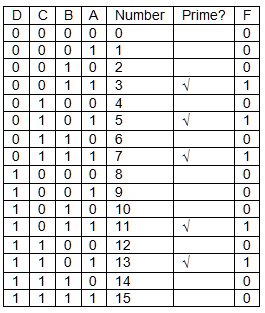Part b.

Obtaining a logical expression

Each of the terms in the truth table is called a minterm and contains each of the four variables in either its true or complemented form. For example, D×C×B×A is a minterm, whereas D×B×A is not a minterm because it contains only three of the four variables.

In this case there are five minterms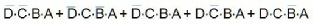The output is true if any of the minterms are true; that is, the output is the Boolean OR of the minterms. Consequently,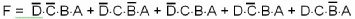Part c.

Generating a Karnaugh Map

We now draw a Karnaugh map for this system. We will step through the creation of a Karnaugh map step-by-step.  Note that this part does not attempt to teach Karnaugh map fundamentals; it helps revise the topic.

Figure 1 illustrates the structure of a 4-variable Karnaugh map. Each of the 16 squares contains a term with four variables, each of which is either in its true form or its complimented form. What is important to note is that adjacent squares (horizontally adjacent or vertically adjacent) differ by one variable only. Each square in the 4-variable Karnaugh map corresponds to one line of the truth table.

Note the sequence along the rows and columns: it’s 00, 01, 11, 10 and not 00, 01, 10, 11 as you might expect. Remember that only one variable changes between adjacent rows and columns.

Figure 1 The 4-variable Karnaugh map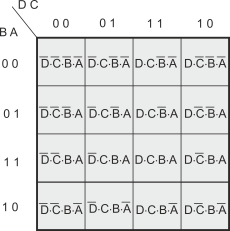Figure 2 illustrates the situation after we have plotted the minterms from the truth table. I have included the number of each square (i.e., the corresponding line in the truth table) in blue in the upper right hand corner in order to make the placing of the minterms clear. Generally, most students don’t write in minterm numbers in this way (but it can sometimes be a big help).

Figure 2 Plotting the minterms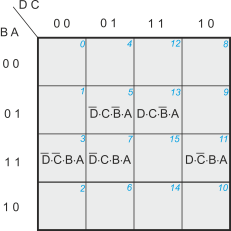Traditionally, the minterms of a Karnaugh map are represented by a 1. All the other squares contain a 0. However, we do not write down the zeroes because they are ‘understood’. Figure 3 shows the Karnaugh map with 1s representing the lines in the truth table with a 1 in the F column.

Figure 3 The Karnaugh map corresponding to the Truth Table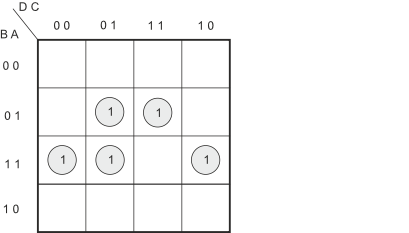The key to solving a Karnaugh map problem is to regroup all the 1s. Sometimes this is not always possible and you can’t rearrange the 1s. If that happens, the Boolean equation cannot be simplified further.

The 1s in a Karnaugh map must be grouped into twos, fours or eights (you can have 16 in a group but that would correspond to F = 1; that is, the function is always true). The groups must be a vertical line or a horizontal line, or a square, or a rectangle. Note that the Karnaugh map is topologically a torus (doughnut) so that the upper and lower edges are adjacent as are the left and right edges. This means that a minterm on the bottom row is adjacent to a minterm on the top row vertically above it. All the four corners of a Karnaugh map are adjacent.

Each 1 on the Karnaugh map MUST be in a group, but it may be shared by several groups; that is, the same element (minterm) may be part of several groupings.

In this case, we can regroup the 1s as figure 4 demonstrates. There are three groups of two 1s; two groups are horizontal and one vertical. Note that one horizontal group (in blue) shares the two edges (because these are actually adjacent). One minterm,  is in two groups.

Figure 4 Forming groups of 1s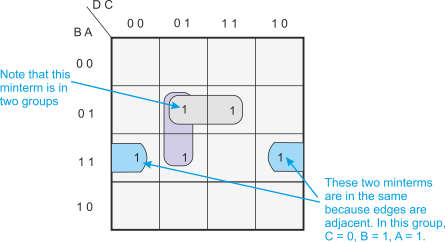We can now write down a simplified expression for F. We ask what variables are common to each group and then sum the three groups. For example, the blue group contains the common elements B = 1, A = 1, C = 0. Note that D is not in this group because it is 0 in the left hand element and 1 in the right hand element.

Figure 5 shows the same Karnaugh maps but with the larger groups relabeled.

Figure 5 Labelling the groups of minterms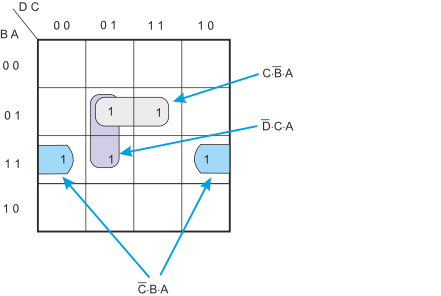Part d.

Generating a Simplified Expression for F

Finally, we can write down the simplified equation for F as: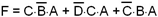As you can see, this is a simpler expression than the original sum of five minterms obtained from the truth table.

Part e

Using Boolean Algebra to Simplify and Expression

Now we need to simplify the original expression, the sum of minterms from the truth table, using Boolean algebra. To do this, we use the rules of Boolean algebra until we cannot simplify the expression further. Doing this takes practice and it is rather difficult to tell whether you have a simplified expression or not. You have to practice solving Boolean equations until you become proficient. With a Karnaugh map, the solution is clear because you either can or cannot form bigger groups or fewer groups. Note that neither Karnaugh maps nor Boolean algebra may lead to a unique solution. Sometime it is possible to express a Boolean equation on more than one way.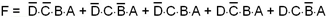We can treat this as conventional algebra and factor the groups; for example,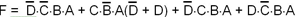Since the sum (logical or) or a variable and its complement is 1, we can rewrite the expression as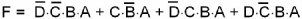We can perform another re-grouping of terms to get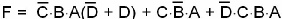Proceeding as before we get: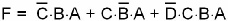Next we re-group again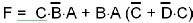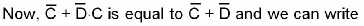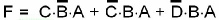Further simplification is not possible. By the way, suppose we compare this with the simplified function from the Karnaugh map; that is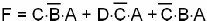Confusingly, these two expressions are not the same but they are logically equivalent. This is because the same Boolean function can sometimes be represented in more than one way (as we pointed out earlier).

Suppose we plot this simplified function on a Karnaugh map. We get the system described by Figure 6. You can see that the 1s on Figure 6 are in exactly the same place as those of Figure 5. The difference is in the way in which the groups are formed. In both cases we have formed the smallest number of large groups. Consequently, both results are correct and equally valid.

Figure 6 The Karnaugh map corresponding to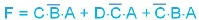Part f.

Designing a Logic Circuit

To create a circuit using AND, OR and NOT gates. We use NOT gates to perform inversion, AND gates for multiplication and OR gates for addition. As in conventional algebra, the multiplication has a higher priority than addition so that X×Y + P×Q must be done as (X×Y) + (P×Q) and not X(Y + P)Q.

Figure 7 illustrates a circuit using AND, OR and NOT gates.

Figure 7 Implementing the simplified function with AND, OR and NOT gates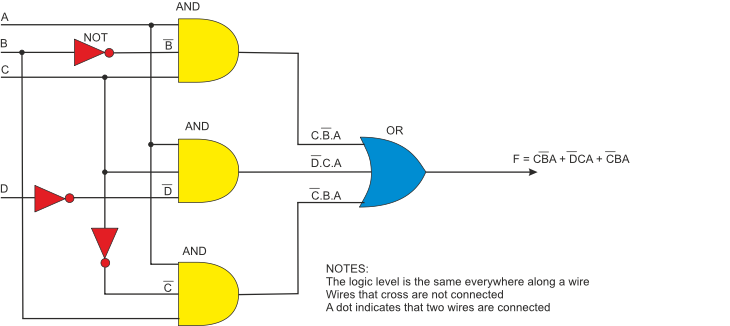Converting and expression in a sum of products form (SoP) is very easy. The SoP form consists of a group of product (AND) terms that are summed (ORed); for example;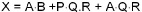Part g

Constructing the circuit with NAND gates only

It is possible to construct any logic circuit from NAND gates only (or NOR gates only) because de Morgan’s theorem provides a means or translating product terms into sum terms, and vice versa. Consider P = Q×R×S

If we invert this expression twice, we get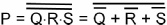Remember that if we complement an expression twice, its value does not change. However, AND terms and changes to OR terms and individual elements are complemented.

In our example we can write: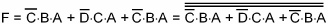We can now apply deMorgan’s theorem to get: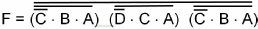Note how we have converted the sum expression into a product expression. Now we are left with only product expressions and inversions.

The transfer function of a 2-input NAND gate is NOT (P AND Q).

If we make Q = R we get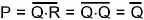Consequently, we can use a NAND gate as a simple inverter.

We can now start constructing the circuit. First note that the entire expression is inverted and we have three terms ANDed. This means that we must use a 3-input NAND gate. Each of the three terms is, itself, a NAND expression. Finally, negated single terms can be generates with a 2-input NAND gate acting as an inverted. Figure 8 illustrates a circuit using NAND gates only.

Figure 8 Implementing the simplified function with NAND gates only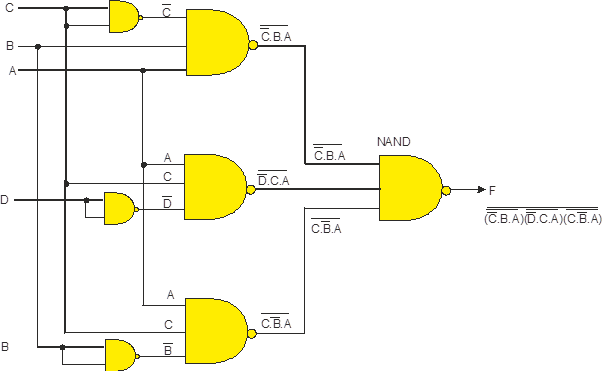Part h

Constructing a Circuit with NOR Gates Only

Designing a circuit with NOR gates only uses the same basic techniques as designing a circuit with NAND gates; that is, the application of deMorgan’s theorem. The only different between NOR gate design and NAND gate design is that the former must eliminate product terms and the latter must eliminate sum terms.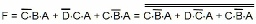Note that the output is just a negated NOR expression. We can do the negation with a 2-input NOR. We are just left with the product terms, C××A, D¬×C×A, and C××A. We can use deMorgan’s theorem again; consider the first term.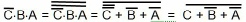Finally, we can construct the NOR-only circuit of figure 10.

Figure 10 A NOR Gate Only Circuit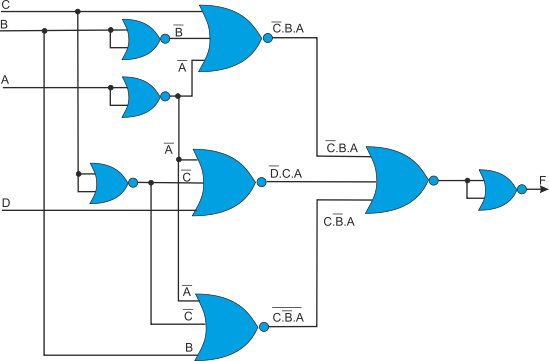Part i

Don’t Care Conditions

Sometimes certain input conditions cannot occur; for example, they may represent physically impossible states or conditions, or choices that are not available. Consequently, if such conditions cannot occur, it does not matter whether we choose those conditions to make the output 0 or 1. However, we can choose the output to be 0 or 1 according to which helps generate the simplest solution.

Consider figure 11 where we’ve plotted the don’t core conditions 0,1,2,3,14,15 are xs in yellow circles.

Figure 11 Don’t Care Conditions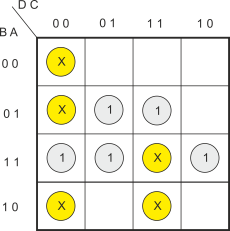If you examine Figure 11 you will find that we can take one of the don’t care conditions (i.e., input represents 15) and create the groups of Figure 12.

Figure 12 Choosing Don’t Care Conditions to Simplify the Karnaugh Map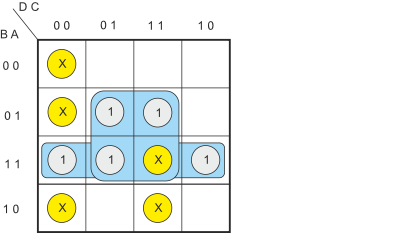In this case the simplified Karnaugh map gives

F = C.A + B.A

This is a considerably simpler expression than the one we originally obtained and can be used to build a less expensive logic circuit with fewer gates (in this case just two 2-input AND gates and a one 2-input OR gate). However, you should appreciate that using don’t care conditions is potentially dangerous because if the impossible input did occur (due to a circuit fault or misuse by the operator), the output would be incorrect.

Part j

Converting Sums of Products into Product of Sums Forms

Converting between SoP and PoS forms invariably means converting AND operations into OR operations and vice versa. The only way of doing this is to use deMorgan’s theorem.

Consider the original simplified expression for F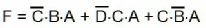We can invert this expression twice as the complement of a complement is the original value; that is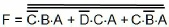We have applied deMorgan’s law using the inner negation to remove the OR operators. Now let’s apply deMorgan’s law a second time to remove the outer negation.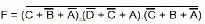We now have the required product of sums form for F. Note that this is not a standard product of sums form because the output is negated. This form would be useful where the output stage of the circuit was a NAND gate.